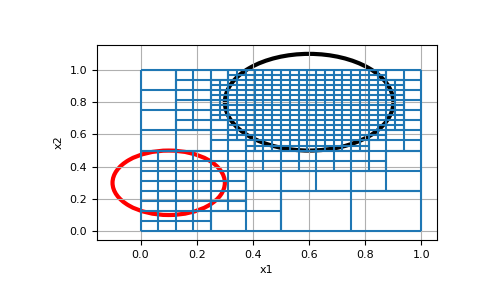# discretize.TreeMesh.refine_ball¶

Refine `TreeMesh` using radial distance (ball) and refinement level for a cluster of points.

For each point in the array points, this method refines the tree mesh based on the radial distance (ball) and refinement level supplied. The method accomplishes this by determining which cells intersect ball(s) and refining them to the prescribed level(s) of refinement.

Parameters
points(`N`, `dim`) array_like

The centers of the refinement balls

radii(`N`) array_like

A 1D array defining the radius for each ball

levels(`N`) array_like `of` `int`

A 1D array defining the maximum refinement level for each ball

finalizebool, `optional`

Whether to finalize after refining

Examples

We create a simple mesh and refine the tree mesh such that all cells that intersect the spherical balls are at the given levels.

```>>> import discretize
>>> import matplotlib.pyplot as plt
>>> import matplotlib.patches as patches
>>> tree_mesh = discretize.TreeMesh([32, 32])
>>> tree_mesh.max_level
5
```

Next we define the center and radius of the two spheres, as well as the level we want to refine them to, and refine the mesh.

```>>> centers = [[0.1, 0.3], [0.6, 0.8]]
>>> levels = [4, 5]
```>>> ax = tree_mesh.plot_grid()